# Analysis of Variance (ANOVA) with RPosted by

In this post we are going to perform an analysis of variance (ANOVA) with R in order to analyze the influences of different variables such as race, education level or job class in the wage.

The data is the same as in the post Descriptive Analysis with R, so you can visit that post in order to get more detail about the data used.

Let’s start the analyis.

Let’s get and transform the data.

``````data<-read.csv2("./Wage.csv",header = TRUE, sep = ",",stringsAsFactors = TRUE)

str(data)``````
``````## 'data.frame':    3000 obs. of  11 variables:
##  \$ year      : int  2006 2004 2003 2003 2005 2008 2009 2008 2006 2004 ...
##  \$ age       : int  18 24 45 43 50 54 44 30 41 52 ...
##  \$ maritl    : Factor w/ 5 levels "1. Never Married",..: 1 1 2 2 4 2 2 1 1 2 ...
##  \$ race      : Factor w/ 4 levels "1. White","2. Black",..: 1 1 1 3 1 1 4 3 2 1 ...
##  \$ education : Factor w/ 5 levels "1. < HS Grad",..: 1 4 3 4 2 4 3 3 3 2 ...
##  \$ region    : Factor w/ 1 level "2. Middle Atlantic": 1 1 1 1 1 1 1 1 1 1 ...
##  \$ jobclass  : Factor w/ 2 levels "1. Industrial",..: 1 2 1 2 2 2 1 2 2 2 ...
##  \$ health    : Factor w/ 2 levels "1. <=Good","2. >=Very Good": 1 2 1 2 1 2 2 1 2 2 ...
##  \$ health_ins: Factor w/ 2 levels "1. Yes","2. No": 2 2 1 1 1 1 1 1 1 1 ...
##  \$ logwage   : Factor w/ 508 levels "3","3.04139268515822",..: 126 105 354 426 126 342 452 287 315 347 ...
##  \$ wage      : Factor w/ 508 levels "100.013486924706",..: 397 376 117 189 397 105 215 50 78 110 ...``````
``````data\$logwage<-as.numeric(as.character(data\$logwage))
data\$wage<-as.numeric(as.character(data\$wage))
str(data)``````
``````## 'data.frame':    3000 obs. of  11 variables:
##  \$ year      : int  2006 2004 2003 2003 2005 2008 2009 2008 2006 2004 ...
##  \$ age       : int  18 24 45 43 50 54 44 30 41 52 ...
##  \$ maritl    : Factor w/ 5 levels "1. Never Married",..: 1 1 2 2 4 2 2 1 1 2 ...
##  \$ race      : Factor w/ 4 levels "1. White","2. Black",..: 1 1 1 3 1 1 4 3 2 1 ...
##  \$ education : Factor w/ 5 levels "1. < HS Grad",..: 1 4 3 4 2 4 3 3 3 2 ...
##  \$ region    : Factor w/ 1 level "2. Middle Atlantic": 1 1 1 1 1 1 1 1 1 1 ...
##  \$ jobclass  : Factor w/ 2 levels "1. Industrial",..: 1 2 1 2 2 2 1 2 2 2 ...
##  \$ health    : Factor w/ 2 levels "1. <=Good","2. >=Very Good": 1 2 1 2 1 2 2 1 2 2 ...
##  \$ health_ins: Factor w/ 2 levels "1. Yes","2. No": 2 2 1 1 1 1 1 1 1 1 ...
##  \$ logwage   : num  4.32 4.26 4.88 5.04 4.32 ...
##  \$ wage      : num  75 70.5 131 154.7 75 ...``````

## One factor analysis of variance (ANOVA)

### Wage and education level

Let’s use ANOVA analysis to determine if there are differences in the wage depending on the education level. We will perform the analysis only for the samples with a wage less than 150k\$.

#### Null and alternative hypotheses

The null hypothesis is that mean wages at all levels of education are equal:

H0: m1=m2=m3=m4=m5

The alternative hypothesis (H1) is that not all means are equal.

#### Modelo

Let’s make the calculation using aov function.

First, we select the data we are interested in:

``````data_wage<-data[data\$wage<150,]
str(data_wage)``````
``````## 'data.frame':    2657 obs. of  11 variables:
##  \$ year      : int  2006 2004 2003 2005 2008 2008 2006 2004 2007 2007 ...
##  \$ age       : int  18 24 45 50 54 30 41 52 45 34 ...
##  \$ maritl    : Factor w/ 5 levels "1. Never Married",..: 1 1 2 4 2 1 1 2 4 2 ...
##  \$ race      : Factor w/ 4 levels "1. White","2. Black",..: 1 1 1 1 1 3 2 1 1 1 ...
##  \$ education : Factor w/ 5 levels "1. < HS Grad",..: 1 4 3 2 4 3 3 2 3 2 ...
##  \$ region    : Factor w/ 1 level "2. Middle Atlantic": 1 1 1 1 1 1 1 1 1 1 ...
##  \$ jobclass  : Factor w/ 2 levels "1. Industrial",..: 1 2 1 2 2 2 2 2 2 1 ...
##  \$ health    : Factor w/ 2 levels "1. <=Good","2. >=Very Good": 1 2 1 1 2 1 2 2 1 2 ...
##  \$ health_ins: Factor w/ 2 levels "1. Yes","2. No": 2 2 1 1 1 1 1 1 1 2 ...
##  \$ logwage   : num  4.32 4.26 4.88 4.32 4.85 ...
##  \$ wage      : num  75 70.5 131 75 127.1 ...``````

Next, we run the function.

``````aov<-aov(wage ~ education, data=data_wage)
summary(aov)``````
``````##               Df  Sum Sq Mean Sq F value Pr(>F)
## education      4  267049   66762   120.5 <2e-16 ***
## Residuals   2652 1468949     554
## ---
## Signif. codes:  0 '***' 0.001 '**' 0.01 '*' 0.05 '.' 0.1 ' ' 1``````

In this case we have a test statistic of 120.5 which yields a rather small p-value, less than 0.05, so we must reject the null hypothesis, therefore the means of the salaries by level of education are not equal, there are differences.

### Model fitting.

Let’s plot the model.

``plot(aov)``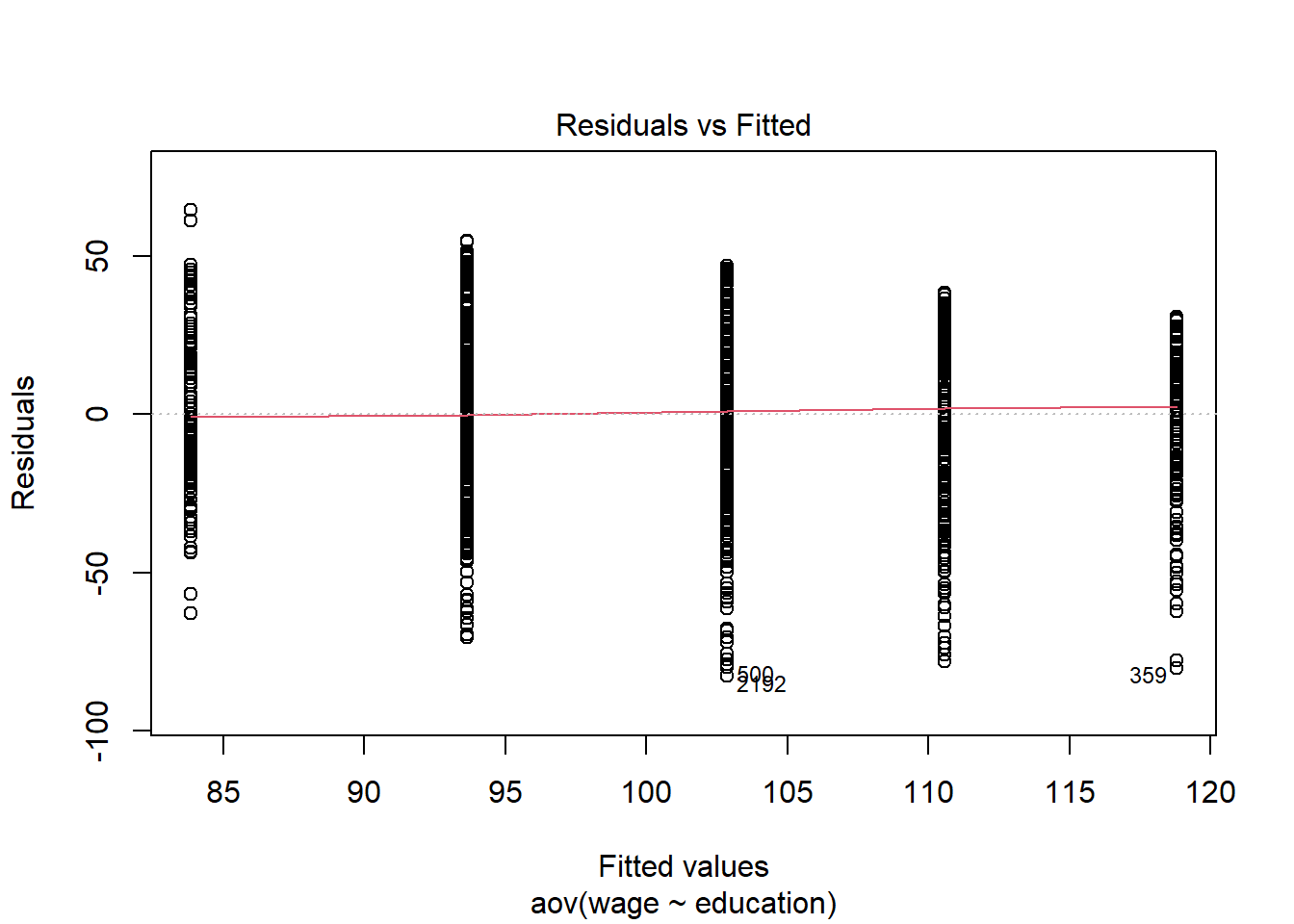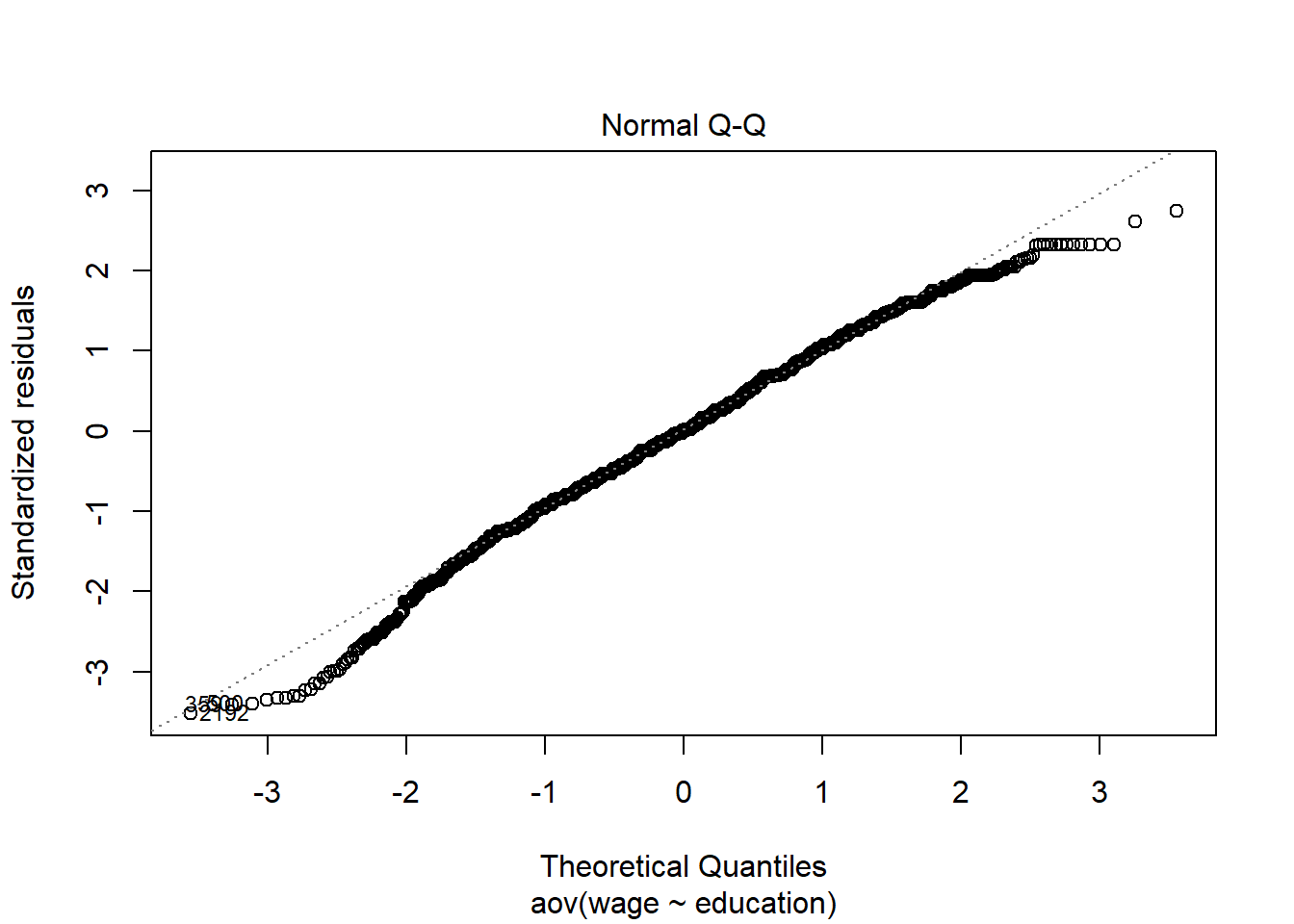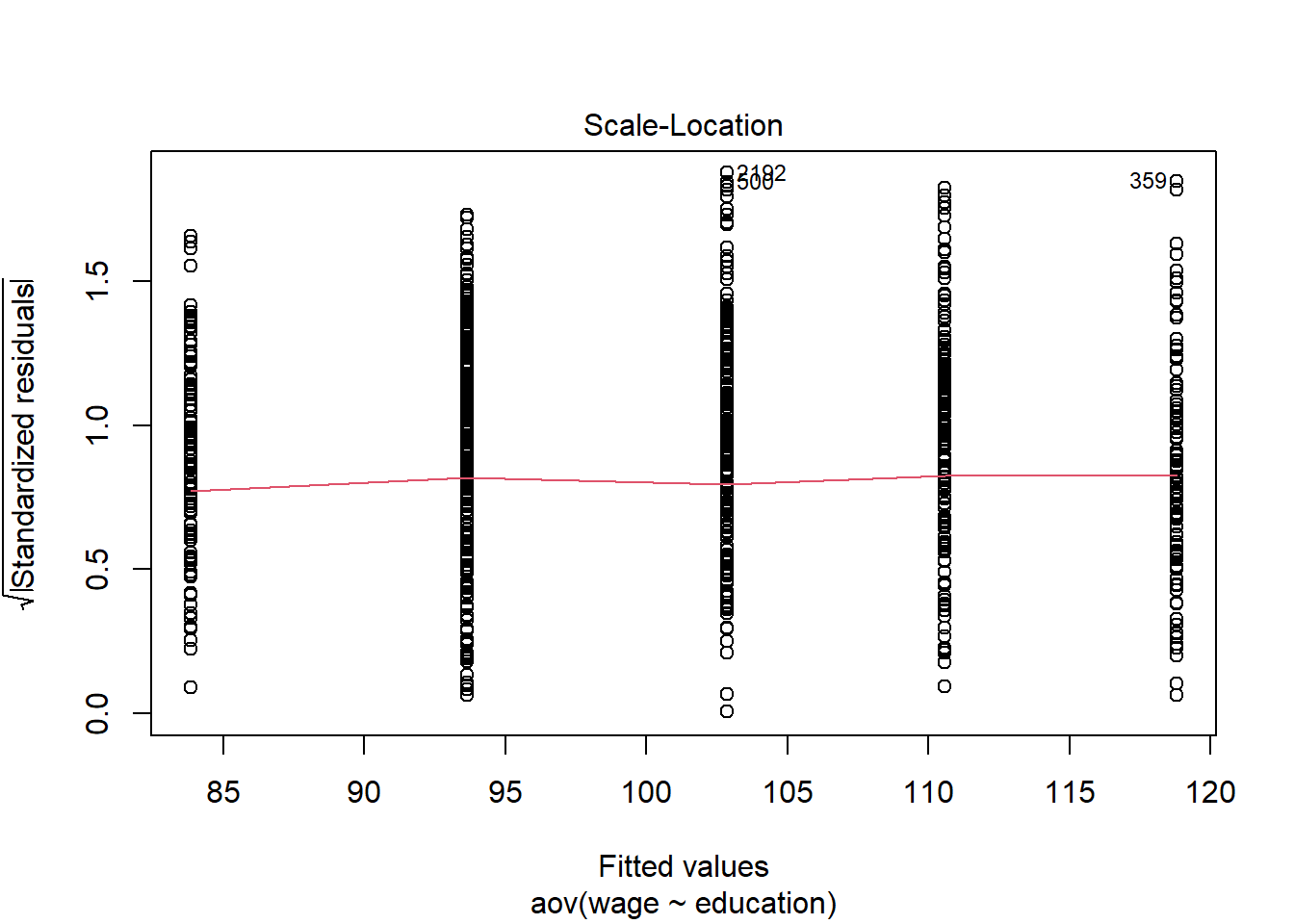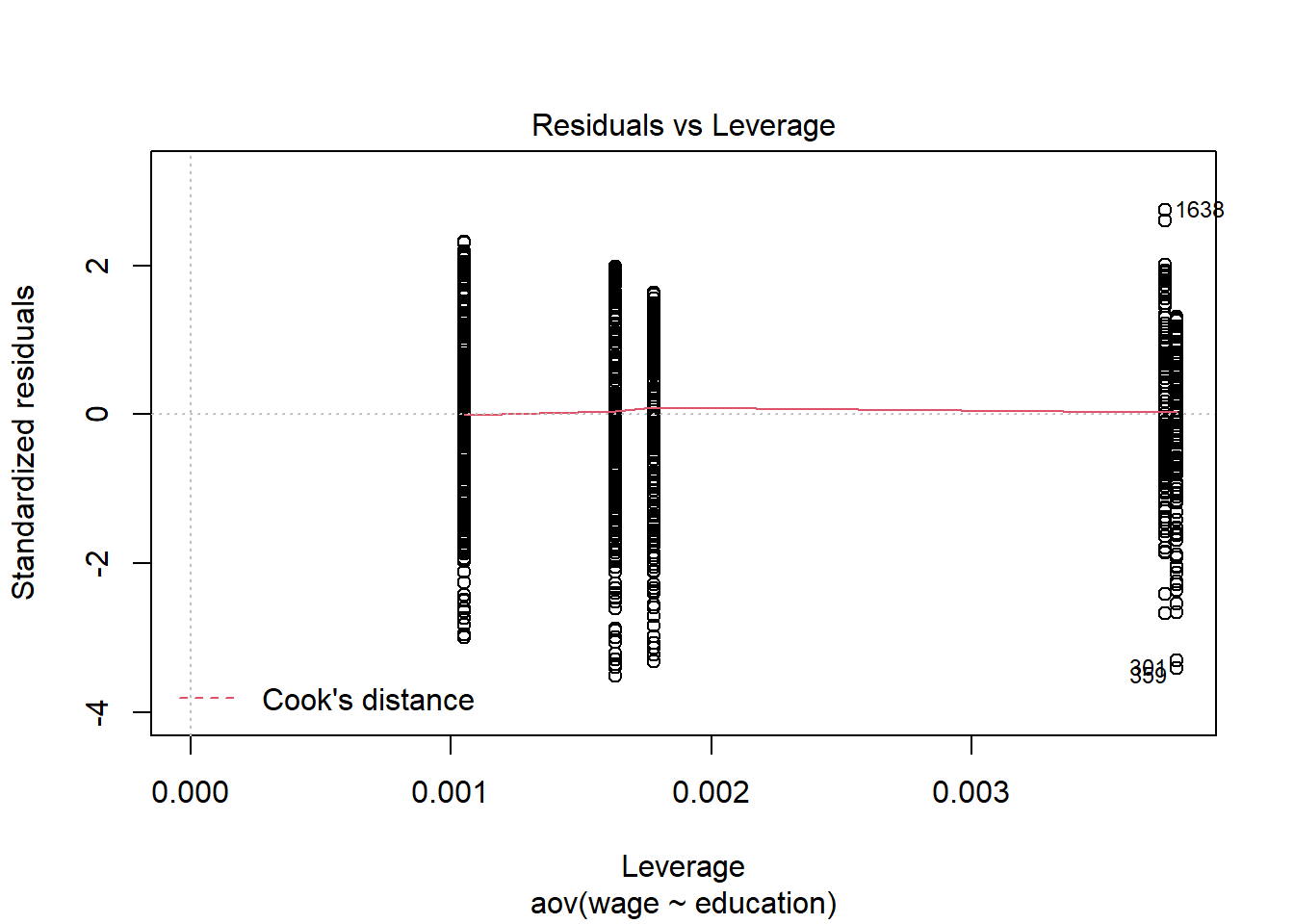We see that the residuals do not follow the line of the normal perfectly although they fit well, except in the exterms, so that given the number of samples, by the central limit theorem, we can consider that the normality condition of ANOVA is fulfilled.

The graph Residuals vs Fitted, shows that the residuals are more or less centered at 0 and no pattern is shown in the points (relationship), also not too many outliers are shown. The parallel lines are due to the fact that we are comparing only 5 categories. Therefore, according to this plot the homoscedasticity condition is fulfilled.

The “Scale-Location” graph shows a similar dispersion in all categories, we can see that the line is more or less straight, so this graph also shows homoelasticity.

On the other hand, the âResiduals vs Leverageâ graph shows that the cook distance is a straight line around zero, so it does not seem that there are values that influence too much the result of the model.

### Non-parametric ANOVA

If the assumptions of normality and homoscedasticity are not met, a nonparametric test such as the Kruskal-Wallis test can be applied.

Let’s applya the Kruskal-Wallis test to check if there are wage differences among races.

First we will prepare the data, we already have in data_wage the salaries < 150K that we are interested in, we will extract from there a dataset with the races that are not “4. Other”.

``````data_race<-data_wage[data_wage\$race!="4. Other",]

str(data_race)``````
``````## 'data.frame':    2621 obs. of  11 variables:
##  \$ year      : int  2006 2004 2003 2005 2008 2008 2006 2004 2007 2007 ...
##  \$ age       : int  18 24 45 50 54 30 41 52 45 34 ...
##  \$ maritl    : Factor w/ 5 levels "1. Never Married",..: 1 1 2 4 2 1 1 2 4 2 ...
##  \$ race      : Factor w/ 4 levels "1. White","2. Black",..: 1 1 1 1 1 3 2 1 1 1 ...
##  \$ education : Factor w/ 5 levels "1. < HS Grad",..: 1 4 3 2 4 3 3 2 3 2 ...
##  \$ region    : Factor w/ 1 level "2. Middle Atlantic": 1 1 1 1 1 1 1 1 1 1 ...
##  \$ jobclass  : Factor w/ 2 levels "1. Industrial",..: 1 2 1 2 2 2 2 2 2 1 ...
##  \$ health    : Factor w/ 2 levels "1. <=Good","2. >=Very Good": 1 2 1 1 2 1 2 2 1 2 ...
##  \$ health_ins: Factor w/ 2 levels "1. Yes","2. No": 2 2 1 1 1 1 1 1 1 2 ...
##  \$ logwage   : num  4.32 4.26 4.88 4.32 4.85 ...
##  \$ wage      : num  75 70.5 131 75 127.1 ...``````
``kruskal.test(wage ~ race, data=data_race)``
``````##
##  Kruskal-Wallis rank sum test
##
## data:  wage by race
## Kruskal-Wallis chi-squared = 11.661, df = 2, p-value = 0.002937``````

The test yields a small p-value, less than 0.05, so we can say that the groups are significantly different from each other, i.e. the pay is not equal for all races.

## Multifactorial ANOVA

let’s look at the effect of race, combined with other factors such as job class and education, on wages. Again, we will use wages under 150k\$ and remove the race ‘4. Other’

### Factors: race and jobclass

We already have the data we need in data_wage, so we perform the analysis.

1. Group the data set.
2. We show the table with the mean for each group.
``````library(dplyr)
group<-group_by(data_race,race,jobclass)
summ<-summarize(group,mean=mean(wage))
summ``````
``````## # A tibble: 6 x 3
## # Groups:   race 
##   race     jobclass        mean
##   <fct>    <fct>          <dbl>
## 1 1. White 1. Industrial   97.7
## 2 1. White 2. Information 106.
## 3 2. Black 1. Industrial   95.1
## 4 2. Black 2. Information  97.8
## 5 3. Asian 1. Industrial   95.2
## 6 3. Asian 2. Information 111.``````
1. Graphics.
``````library(ggplot2)
library(ggthemes)
pd <- position_dodge(width = 0.2)

ggplot(data=summ,aes(x=race,y=mean,group=jobclass,colour=jobclass))+geom_line(aes(linetype=jobclass),position=pd)+
geom_point(size=3,position=pd,color="black") ``````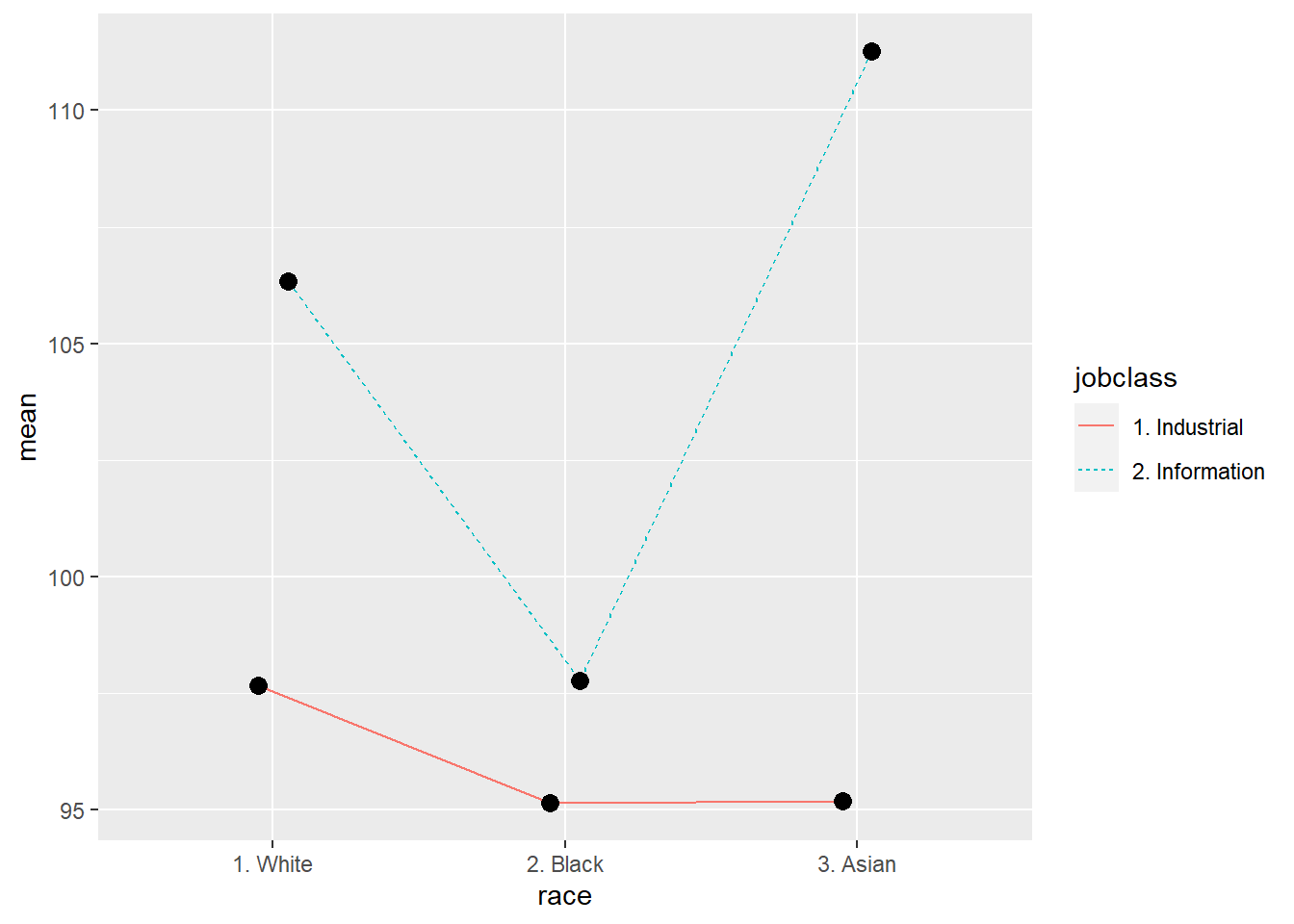In the graph we can see that there is a main effect of the type of work with respect to the mean salary, since the lines are not parallel nor do they follow the same slopes, in addition, both lines change slope at each point, which indicates that there is also a main effect of race with respect to the mean salary. It is also shown that there is an interaction between type of work and race since the points are different in each combination of these variables and no parallelism is observed between the two lines.

#### ANOVA Model

Let’s apply ANOVA model to check if the wage varies among the different conditions.

``````aov<-aov(wage ~ race*jobclass, data=data_race)
summary(aov)``````
``````##                 Df  Sum Sq Mean Sq F value  Pr(>F)
## race             2    6176    3088   4.898 0.00753 **
## jobclass         1   46347   46347  73.515 < 2e-16 ***
## race:jobclass    2    4489    2245   3.560 0.02857 *
## Residuals     2615 1648621     630
## ---
## Signif. codes:  0 '***' 0.001 '**' 0.01 '*' 0.05 '.' 0.1 ' ' 1``````

According to the results of the ANOVA model, we can observe that the F statistic is below 0.05 in all rows, this means that effectively, as we saw in the graphs, both variables are statistically significant, that is to say that there are differences in salary with respect to race, there are also differences with respect to the type of work, and in addition we can say that there is an interaction between race and type of work with respect to salary.

#### Model fitting

Let’s show the graphics.

``plot(aov)``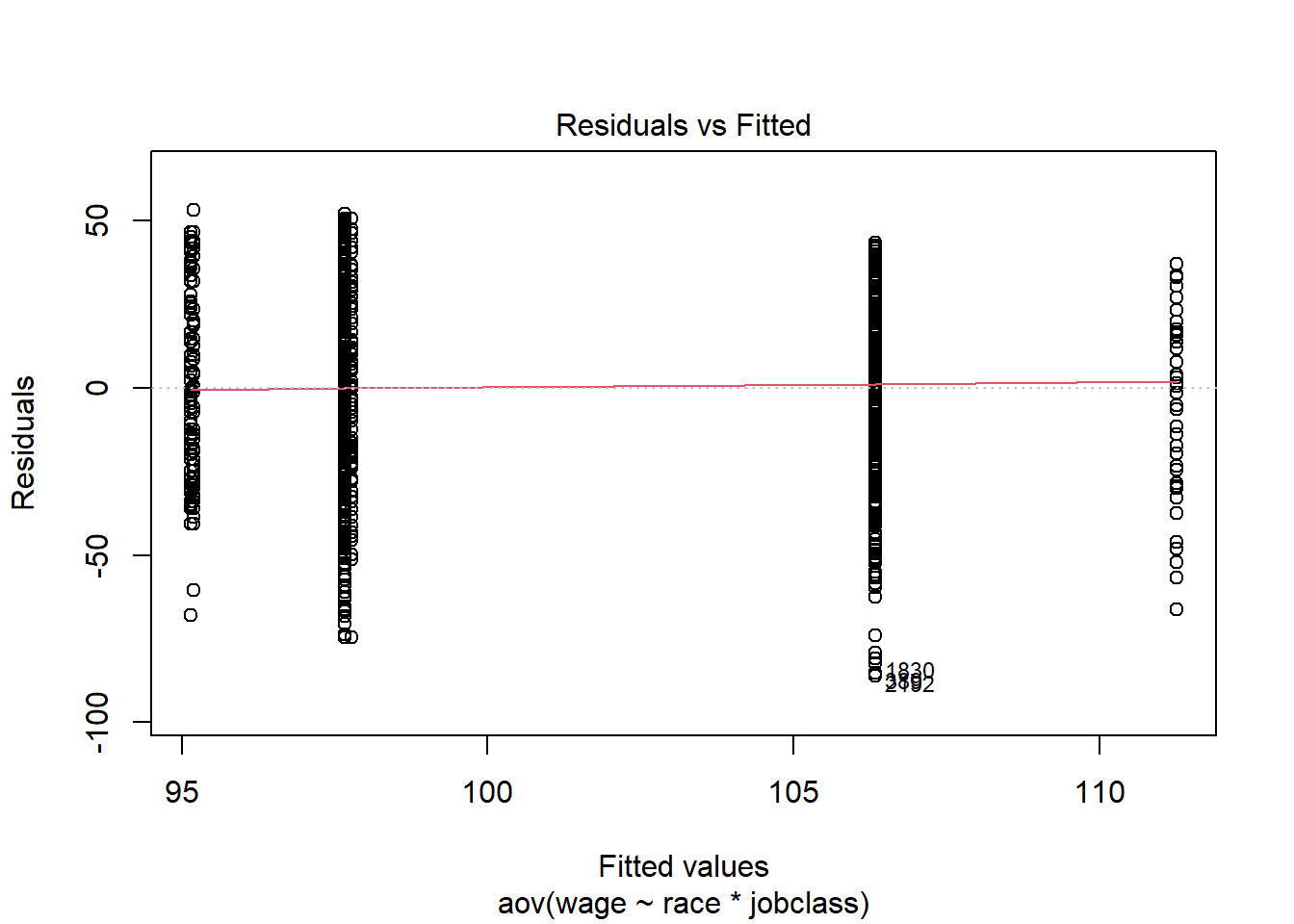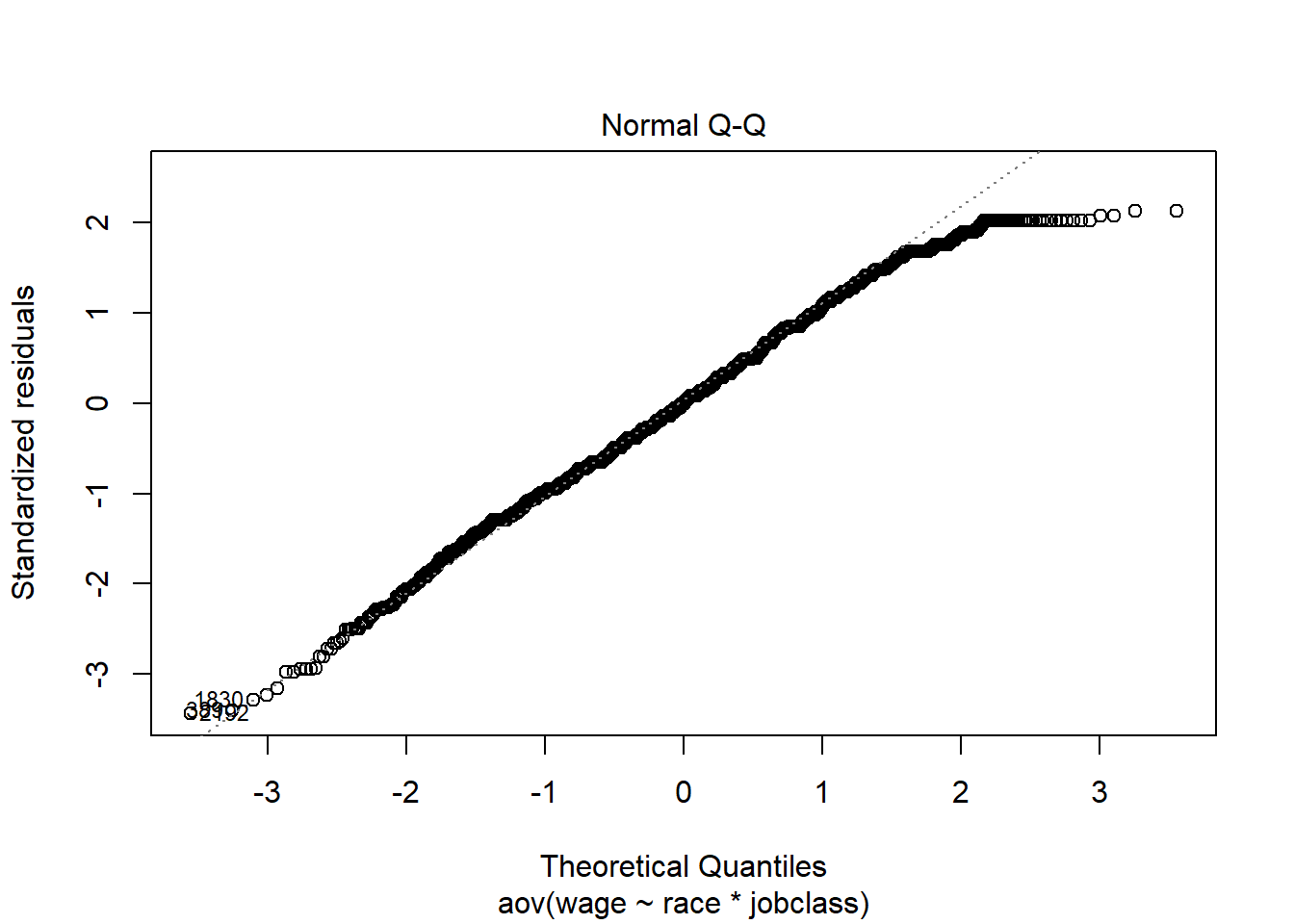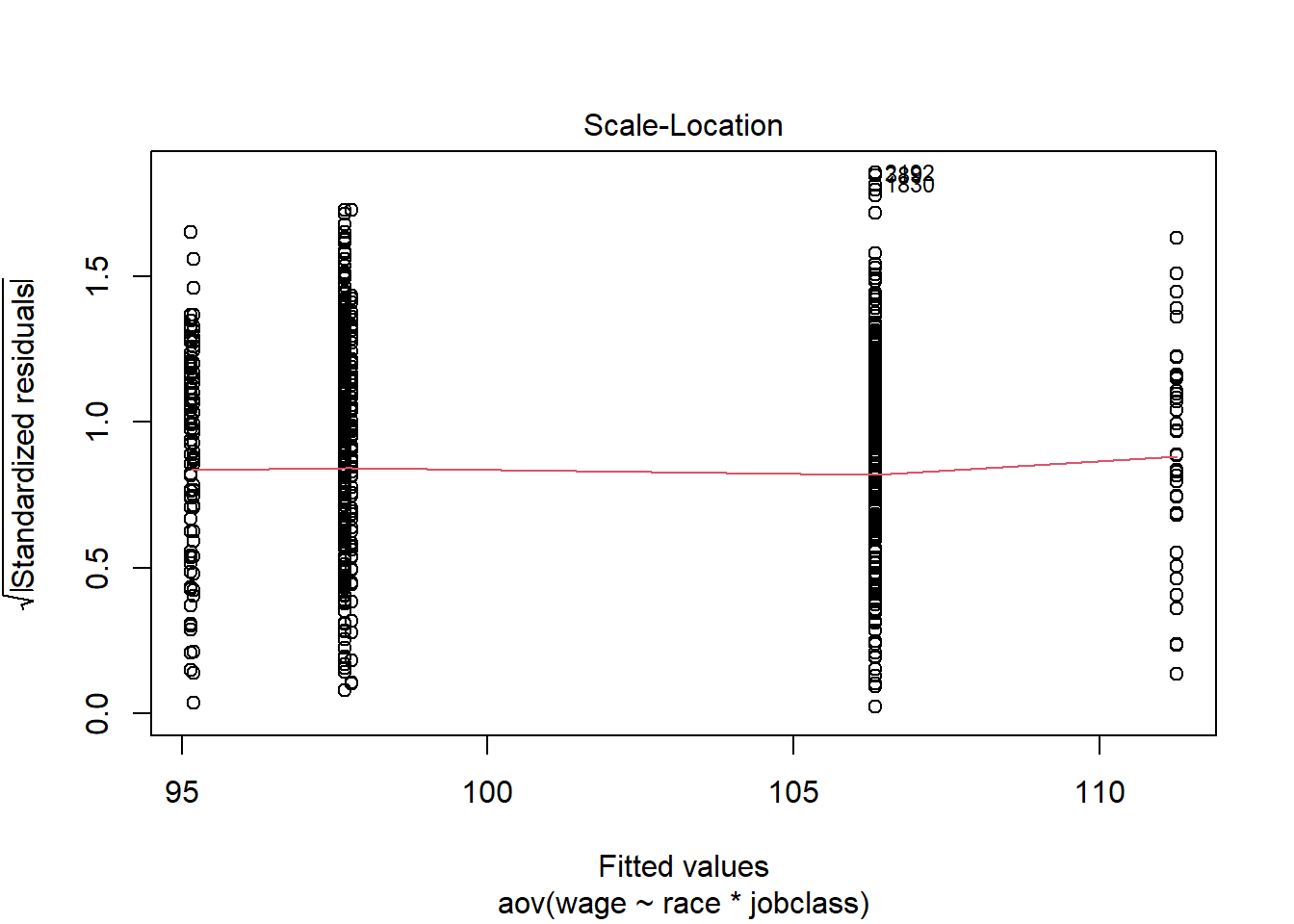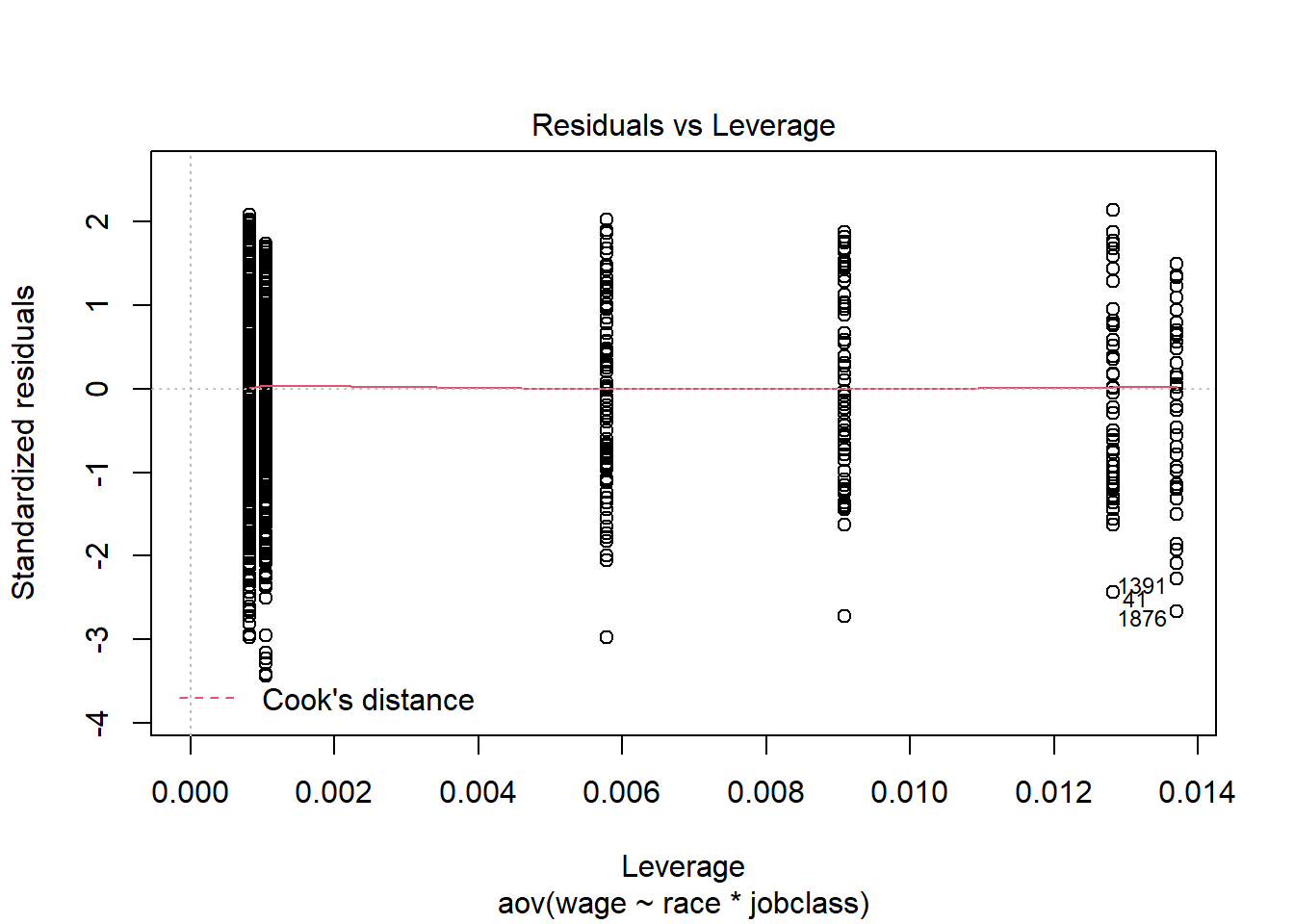Looking at the Normal Q-Q plot, we observe that the residuals points fit well to the normal line, deviating at the upper end, therefore, taking into account the central limit theorem we can say that the normality condition is fulfilled.

The âResiduals vs Fittedâ plot, shows that the residuals points are more or less centered at 0 and no pattern is shown in the points, also not too many outliers are shown. The parallel lines are due to the fact that we are comparing few categories. Therefore, according to this plot the homoscedasticity condition is fulfilled.

The “Scale-Location” graph shows a similar dispersion in all categories, we can see that the line is more or less straight, so this graph also shows homoelasticity.

On the other hand, the “Residuals vs Leverage” graph shows that the cook’s distance is practically 0, so it does not seem that there are values that influence too much the result of the model.

### Race and education level

Let’s start grouping data and showing the table.

``````library(dplyr)
group<-group_by(data_race,race,education)
summ<-summarize(group,mean=mean(wage))
summ``````
``````## # A tibble: 15 x 3
## # Groups:   race 
##    race     education           mean
##    <fct>    <fct>              <dbl>
##  1 1. White 1. < HS Grad        84.1
##  2 1. White 2. HS Grad          94.8
##  3 1. White 3. Some College    104.
##  4 1. White 4. College Grad    111.
##  5 1. White 5. Advanced Degree 119.
##  6 2. Black 1. < HS Grad        86.8
##  7 2. Black 2. HS Grad          89.3
##  8 2. Black 3. Some College     98.5
##  9 2. Black 4. College Grad    109.
## 10 2. Black 5. Advanced Degree 117.
## 11 3. Asian 1. < HS Grad        75.1
## 12 3. Asian 2. HS Grad          82.6
## 13 3. Asian 3. Some College    100.
## 14 3. Asian 4. College Grad    113.
## 15 3. Asian 5. Advanced Degree 119.``````

Let’s analyze the graphics.

``````library(ggplot2)
library(ggthemes)
pd <- position_dodge(width = 0.2)

ggplot(data=summ,aes(x=race,y=mean,group=education,colour=education))+geom_line(aes(linetype=education),position=pd)+
geom_point(size=3,position=pd,color="black") ``````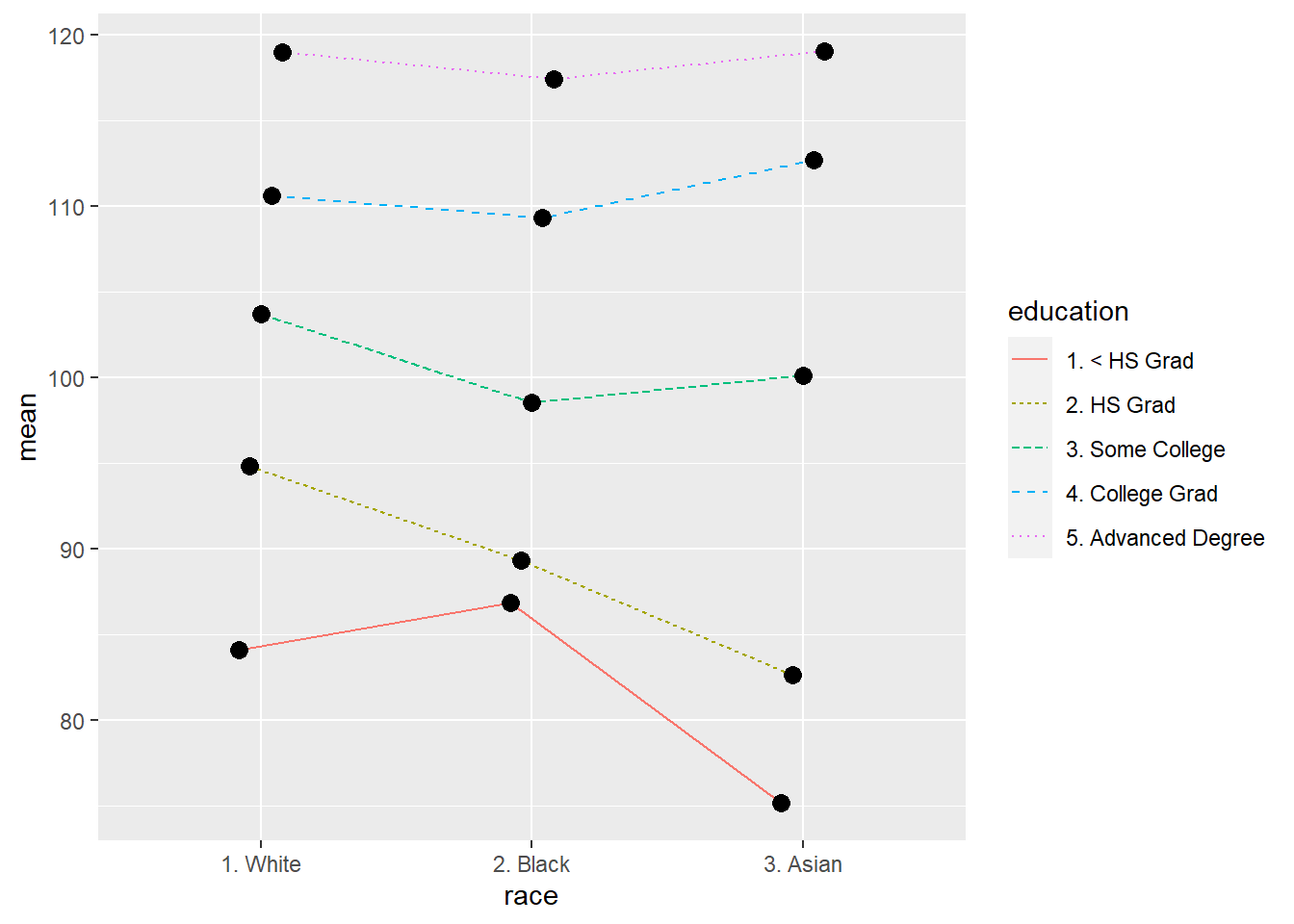We can see that the lines change slope at each point, which indicates that there is an effect of race on the mean wage, and the fact that the lines are separated indicates that there is also an effect of educational level on wage. As for the interaction effect we see that for the 3 higher levels of education there is little interaction between the variables with respect to salary, since the lines are almost parallel, this interaction effect is somewhat more pronounced in the lower educational levels (<HS Grad and HS Grad) since we see that their shape varies more than the lines between the 3 higher levels.

#### ANOVA model.

``````aov2<-aov(wage ~ race*education, data=data_race)
summary(aov2)``````
``````##                  Df  Sum Sq Mean Sq F value  Pr(>F)
## race              2    6176    3088   5.615 0.00369 **
## education         4  259984   64996 118.180 < 2e-16 ***
## race:education    8    6241     780   1.418 0.18340
## Residuals      2606 1433232     550
## ---
## Signif. codes:  0 '***' 0.001 '**' 0.01 '*' 0.05 '.' 0.1 ' ' 1``````

We observe, according to the F statistic, that both race and educational level individually have an effect on salary, since this statistic is less than 0.05, however, the interaction statistic does not show statistical significance, so we cannot affirm that there is an interaction between race and educational level with respect to salary.

#### Model fitting

Let’s show the graphs, adding a graph with Cook distances.

``plot(aov2)``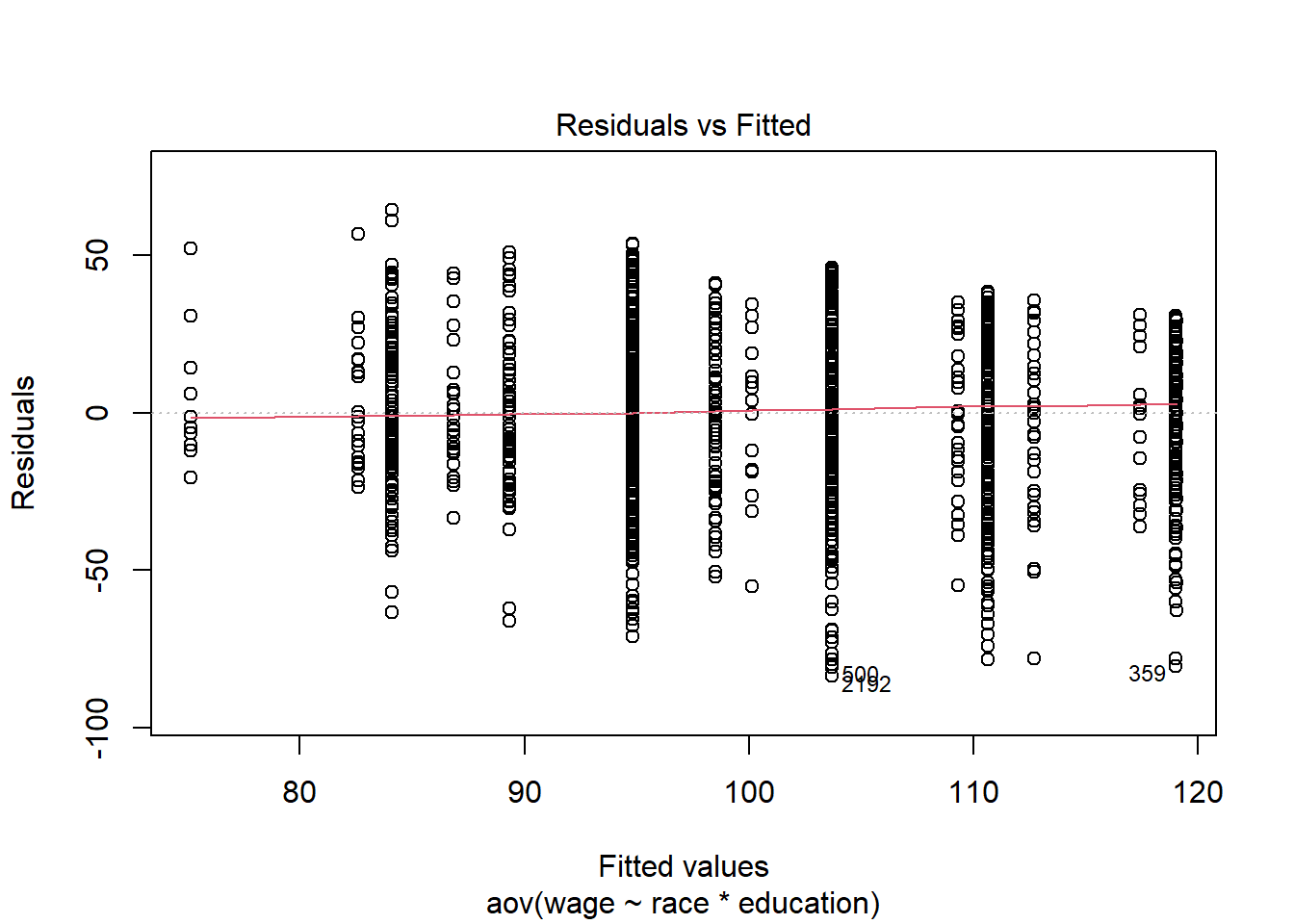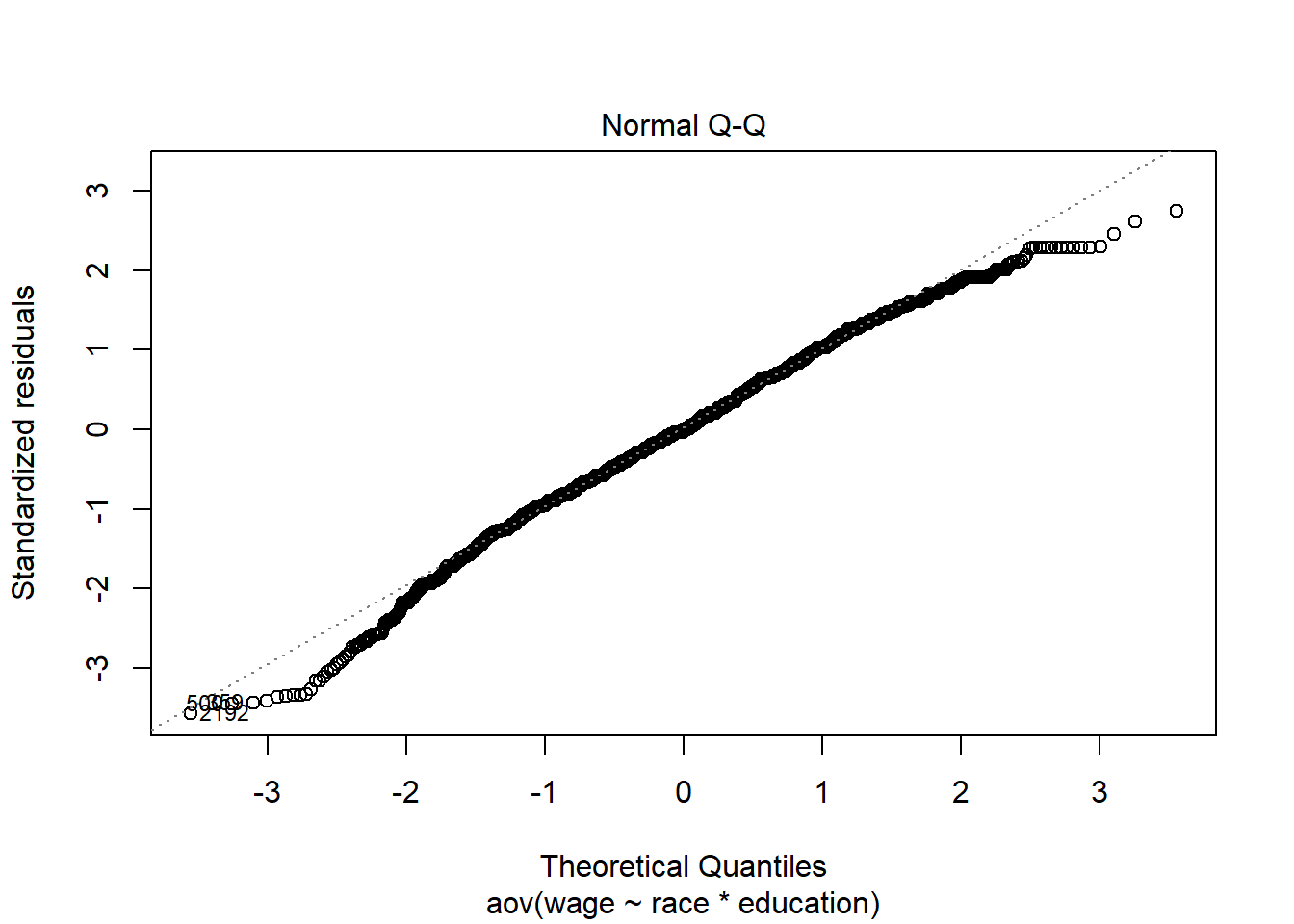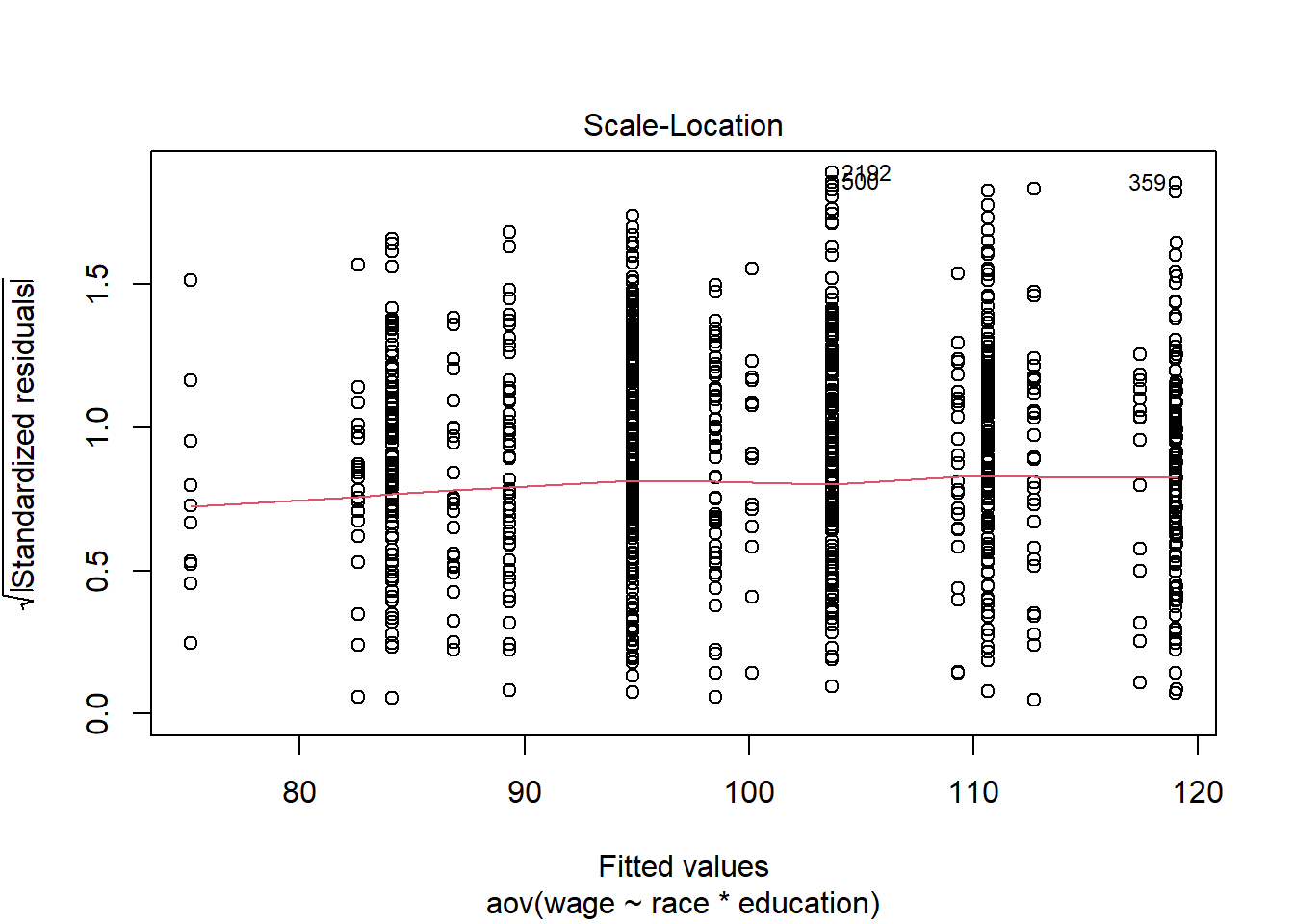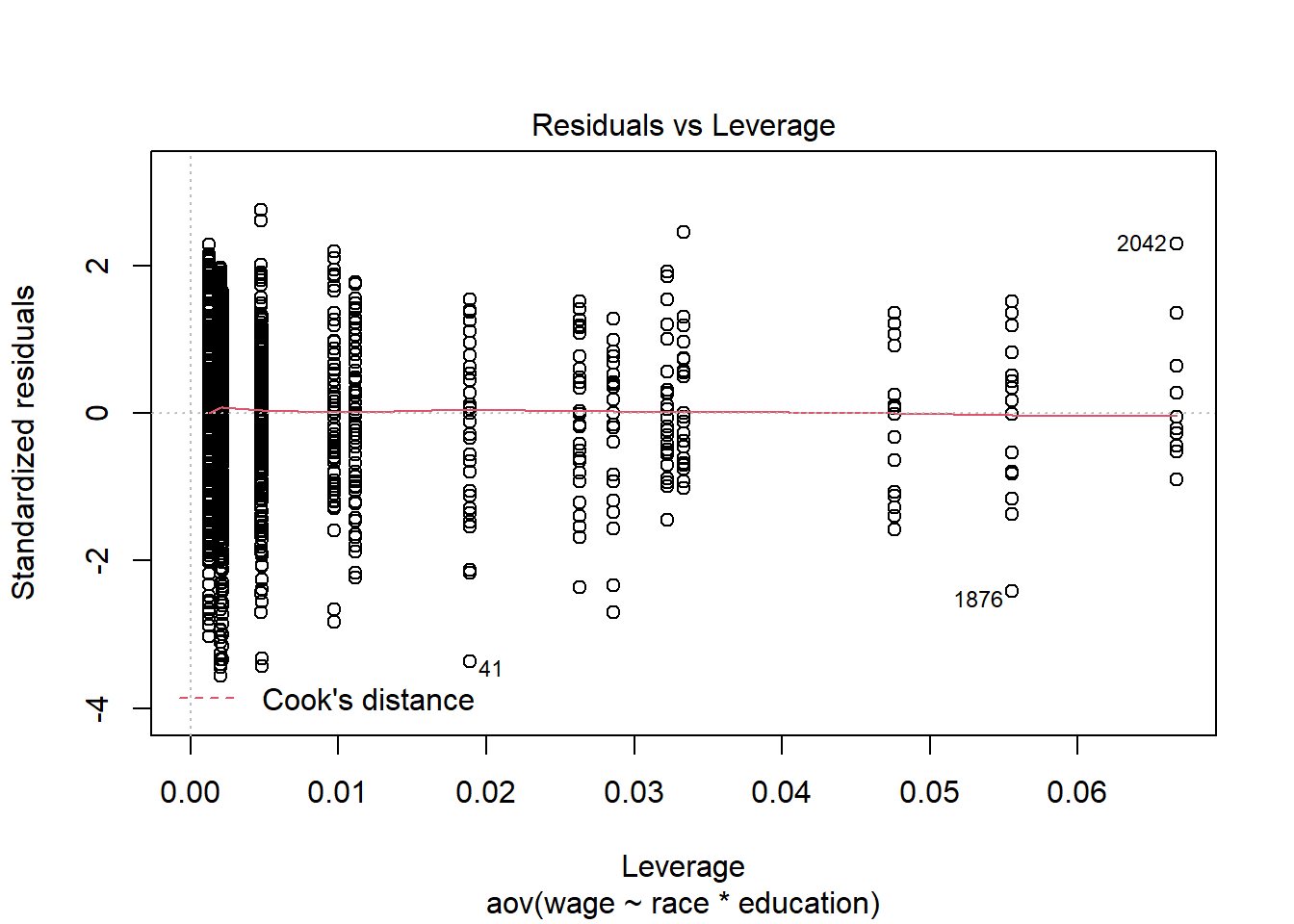``plot(aov2, which=4, cook.levels=cutoff)``